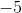## Example Questions

← Previous 1

### Example Question #1 : How To Solve For A Variable As Part Of A Fraction

If  (t-5)/2t =13/19, what is the value of t?

80/19

95/7

(-65)/25

(-95)/7

(-95)/7

Explanation:

We start by cross multiplying to get the equation 26t=19t-95. We then subtract 19t from 26t giving us 7t= -95. We then divide by 7, giving us t= (-95)/7.

### Example Question #2 : How To Solve For A Variable As Part Of A Fraction

If x/3 = 50, then what is x/10 equal to?

5

15

150

1500

50

15

Explanation:

1. Solve for x in x/3 = 50

2. = 150

3.Substitute 150 for x in x/10

4. x = 15

### Example Question #3 : How To Solve For A Variable As Part Of A Fraction

There are m number of people on a deserted island. They drink n liters of water a day. There are x number of 10 liter bottles on the island. On what day will they run out of water?

n/10xm

10mn/x

m/10xn

10x/mn

10/nxm

10x/mn

Explanation:

There are only x * 10 liters of water on the island. n * m equals how many liters are consumed per day. Divide x * 10 liters by n * m. Note the units (days) are correct in the answer.

### Example Question #4 : How To Solve For A Variable As Part Of A Fraction

The maximum number of sweaters that Lauren can sew every day is equal to s, and the amount, in cents, that she charges for each sweater is equal to c. Which of the following expressions is equivalent to the maximum amount of money that Lauren can make, in dollars, after three weeks?

2100sc

3c/(100s)

300c/s

2100s/c

21sc/100

21sc/100

Explanation:

The amount of money that Lauren can make depends on the number of sweaters that she can make. If she makes at most s sweaters a day, then we can multiply the number of days that she works by s to determine the total number of sweaters she makes.

total number of sweaters = (s)(number of days)

We are told to consider a time interval of three weeks. Because there are seven days in one week, the number of days over this period of time would equal 3(7), or 21 days. In other words, there are 21 days in three weeks Thus, the number of sweaters is equal to the product of s and 21.

total number of sweaters = (s)(21)

Now that we have the number of sweaters Lauren can make, we can multiply this by the cost of each sweater, which is equal to c cents, in order to obtain the amount of money she eared.

amount of money earned = (number of sweaters)(cost of each sweater)

amount of money earned = s(21)(c)

However, because the price of each sweater is given in terms of cents, the amount of money s(21)(c) will be equal to the number of cents she makes. The question, though, asks us to find the amount of money in dollars. We must use a conversion factor to change the number of cents to dollars. Remember that there are 100 cents per dollar.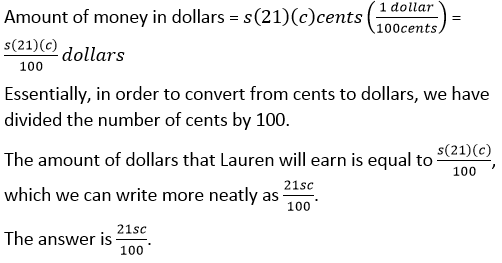### Example Question #1151 : Algebra

If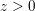, what is 40 percent of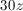?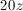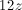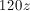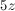Explanation:

To find 40 percent ofmultiply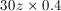The result is### Example Question #1152 : Algebra

Solve for.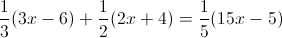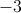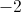Explanation:

First distribute the fractions: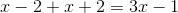Combine like terms: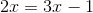### Example Question #1153 : Algebra

Solve for.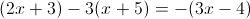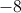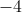Explanation:

First distribute to eliminate the parentheses: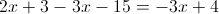Then combine like terms: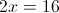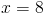### Example Question #1154 : Algebra

x = 1/2

What does 1/x + 1/(x + 4) equal?

4

20/9

9/2

20

4/9

20/9

Explanation:

1/x + 1/(x+4) =

1/(1/2) + 1/ (1/2 + 4) =

1/ (1/2) + 1 / (9/2)  =

2 + 2/9

20/9

### Example Question #1 : How To Solve For A Variable As Part Of A Fraction

Solve for.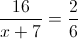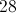Explanation:

Cross multiply.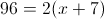Dsitribute.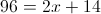Solve for.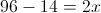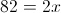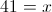### Example Question #1155 : Algebra

If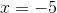, what is the value of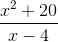?